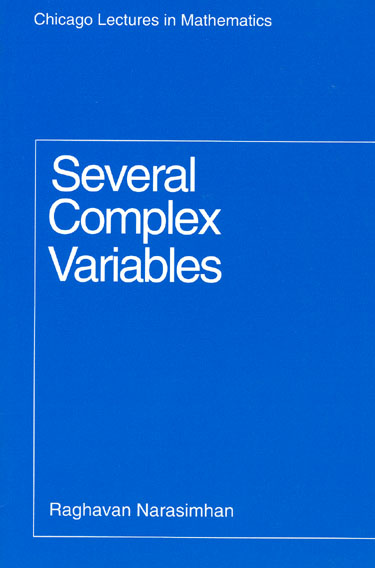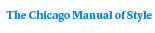# Several Complex Variables

Raghavan Narasimhan184 pages | 5-1/4 x 8 | © 1971
Paper \$46.00 ISBN: 9780226568171 Published February 1995
Drawn from lectures given by Raghavan Narasimhan at the University of Geneva and the University of Chicago, this book presents the part of the theory of several complex variables pertaining to unramified domains over C . Topics discussed are Hartogs’ theory, domains in holomorphy, and automorphism of bounded domains.
Table of Contents• Contents
Contents
PREFACE
CHAPTER 1. ELEMENTARY PROPERTIES OF FUNCTIONS OF SEVERAL COMPLEX VARIABLES
Notations. Holomorphic functions. Cauchy’s formula and some consequences. The open mapping theorem.
Weierstrass’ and Montel’s theorems.
CHAPTER 2. ANALYTIC CONTINUATION: ELEMENTARY THEORY
Extension of holomorphic functions from the boundary of a polydisc. Reinhardt domains.
CHAPTER 3. SUBHARMONIC FUNCTIONS AND HARTOGS’ THEOREM
Definition and basic properties of harmonic and subharmonic functions. Some examples and applications. Hartogs’ theorem on separate analyticity. Exceptional sets of subharmonic
functions.
CHAPTER 4. HARTOGS’ THEOREM ON THE SINGULARITIES OF HOLOMORPHIC FUNCTIONS
Analytic sets. The Riemann continuation theorem. Rado’s theorem. Hartogs’ continuity theorem. Properties of the Hartogs radius. Analyticity of certain singular sets.
CHAPTER 5. AUTOMORPHISMS OF BOUNDED DOMAINS
Cartan’s uniqueness theorem. Automorphisms of circular domains, in particular of polydiscs. Poincare’s theorem that the polydisc and the ball are analytically distinct. Proper holomorphic maps. A theorem of Remmert-Stein and some generalizations.  Limits of automorphisms: Cartan’s theorem. Action of Aut(D) on D, finite generation of some discrete groups. An injective holomorphic map from  D C Cn into Cn is an isomorphism.
CHAPTER 6. ANALYTIC CONTINUATION: ENVELOPES OF HOLOMORPHY
S- extension of a domain over ~. Envelope of holomorphy: basic properties. Examples: The envelope of a domain in C   may no longer be in (C; the envelope of a domain not in n  n
C   may be in ~.
CHAPTER 7. DOMAINS OF HOLOMORPHY: CONVEXITY THEORY
Holomorph-convexity. Properties of the distance to the boundary. First main theorem of Cartan-Thullen. Second main theorem of Cartan-Thullen. Applications and examples:
products, Reinhardt domains and tubes.  D is a domain of holomorphy if D/Aut(D) is compact.
CHAPTER 8. DOMAINS OF HOLOMORPHY: OKA’S THEOREM
Hadamard’s three domains theorem and Schwarz’s lemma.
Behavior of normalized polynomials. Bishop’s proof of Oka’s theorem.
CHAPTER 9. AUTOMORPHISMS OF BOUNDED DOMAINS: CARTAN’S THEOREM
Vector fields and Lie’s theorem: Existence of the local Lie group corresponding to a Lie algebra of vector fields. Cartan’s theorem: vector fields associated to Aut(D),
finite dimensionality of the space of vector fields associated to Aut(D). Existence of vector fields associated to Aut(D).
Proof of Cartan’s theorem.
REFERENCES